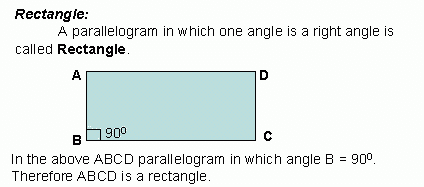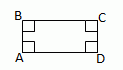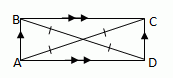Name: ___________________Date:___________________Properties of Rectangle: Opposite sides of a rectangle are equal or congruent. Opposite angles of a rectangle are equal. All its angles are right angles; or The diagonals of a rectangle bisect each other. Each diagonal divides the rectangle into two congruent triangles. The diagonals of a rectangle are equal. Both pairs of opposite sides are equal and one angle is 90¯; or Both pairs of opposite sides are equal and its diagonals are equal. THEOREMS A quadrilateral is a rectangle if and only if it has four right angles.ÅA, ÅB, ÅC, ÅD are 900 A parallelogram is a rectangle, if and only if its diagonals are congruent.AC @ BD Directions: Read the above review points carefully and answer the following questions: Illustrate each of the above review points by drawing a Rectangle. Explain the different properties of a Rectangle, in your own words, with examples. Lilly measures her 10 cm x 6 cm garden plot. She found that all its edges are of angle measure 90¯. What will be the shape of her garden? Give reasons. What will be the value of one of the angles of a rectangle if one side is given by 17 cm and other side by 12 cm? Give reasons. If the lengths of the rectangle is x+31 and 5x-9 what is its length. For the following rectangle is the diagonal AC=10, find the mÅDOA, mÅBOC, BO, OD, AO, OC, ACName: ___________________Date:___________________